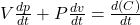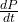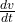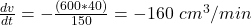## Boyle’s Law states that when a sample of gas is compressed at a constant temperature, the pressure P and volume V satisfy the equation PV =

Question

Boyle’s Law states that when a sample of gas is compressed at a constant temperature, the pressure P and volume V satisfy the equation PV = C, where C is a constant. Suppose that at a certain instant the volume is 600 cm3, the pressure is 150 kPa, and the pressure is increasing at a rate of 40 kPa/min. At what rate is the volume decreasing at this instant?

in progress 0
3 months 2021-07-19T22:36:52+00:00 1 Answers 178 views 0

The volume is decreasing at 160 cm³/min

Explanation:

Given;

Boyle’s law,  PV = C

where;

P is pressure of the gas

V is volume of the gas

C is constant

Differentiate this equation using product rule:Given;(increasing pressure rate of the gas) = 40 kPa/min

V (volume of the gas) =  600 cm³

P (pressure of the gas) = 150 kPa

Substitute in these values in the differential equation above and calculate the rate at which the volume is decreasing ();

(600 x 40) + (150 x) = 0Therefore, the volume is decreasing at 160 cm³/min